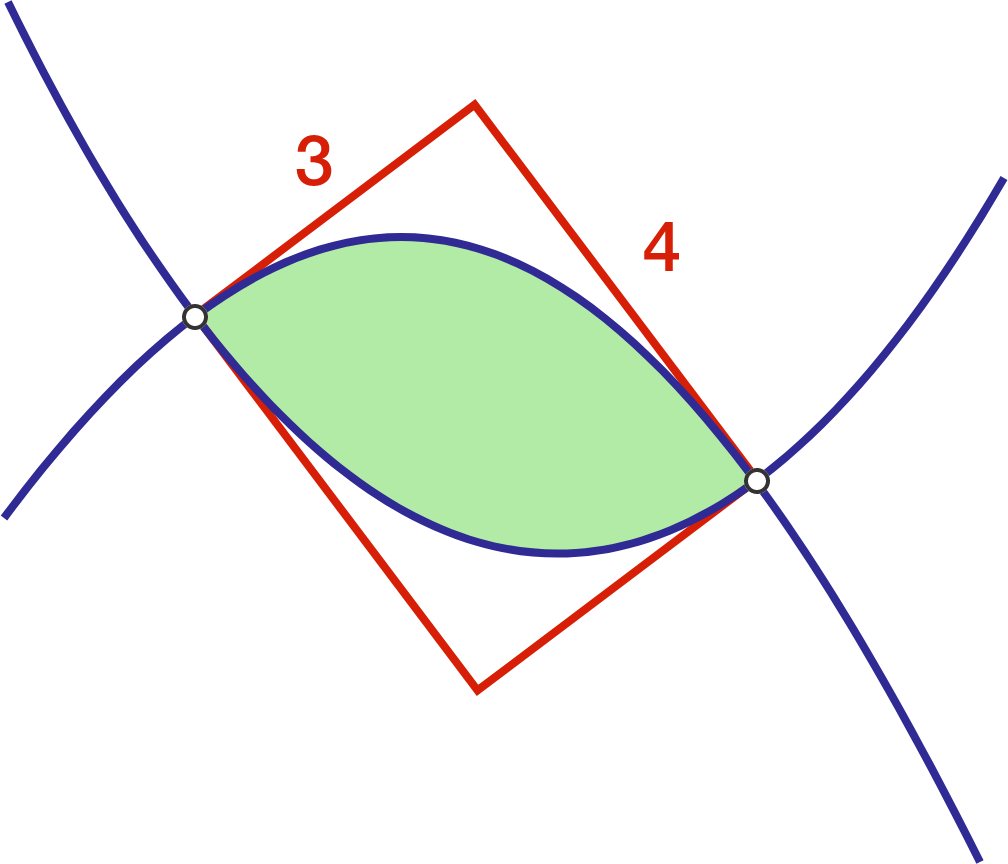# Boxed parabolas

Calculus Level 2

Two parabolas (in blue) intersect at two points. The four tangent lines (in red) at their points of intersection form a $3\times 4$ rectangle. Find the area of the closed region created by both parabolas.Find the area of the region bounded by the 2 curves.

×